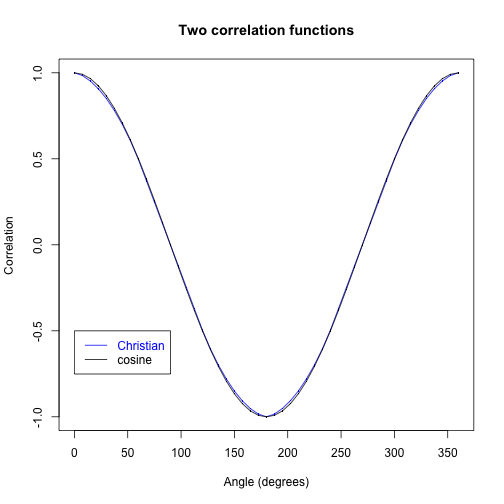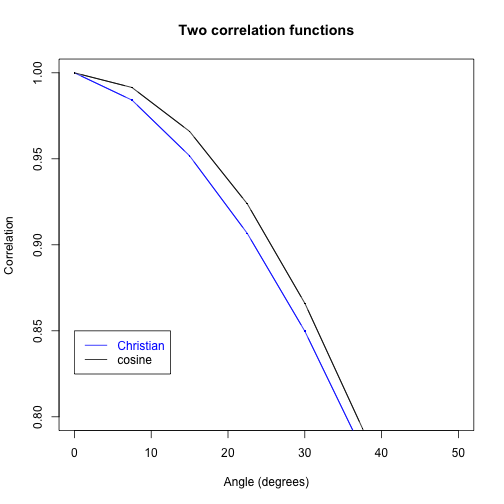### EPRB2.R

richard — Mar 1, 2014, 11:42 PM

``````## Simulation of the "Christian 2.0" model for the EPR-B correlations,
## S^1 version (aka 2-D: think of S^1 as subset of R^2)

## "Christian 2.0" refers to the second edition of Joy Christian's book, see
## http://libertesphilosophica.info/blog/

## This is an implementation (by RDG) of Michel Fodje's algorithm:
## https://github.com/minkwe/epr-simple/

set.seed(8765)

## For reproducibility. Replace integer seed by
## dream up one for you in its own mysterious way (system time + process ID).

## Measurement angles for setting "a": directions in the equatorial plane

angles <- seq(from = 0, to = 360, by = 7.5) * 2 * pi / 360

K <- length(angles)
corrs <- numeric(K)   ## Container for correlations
Ns <- numeric(K)     ## Container for number of states

beta <- 0 * 2 * pi / 360  ## Measurement direction "b" fixed, in equatorial plane

M <- 10^6   ## Sample size. Next, try 10^7 or even 10^8 ...

## I use the same, single sample of "M" realizations of hidden states
## for all measurement directions.
## This saves a lot of time, and reduces variance when we look at *differences*

t <- runif(M, 0, 2*pi)
x <- cos(t)
y <- sin(t)
e <- rbind(x, y)  ## 2 x M matrix
## The M columns of e represent M uniform random points on the sphere S^1

theta <- runif(M, 0, pi/2)  ## Joy and Minkwe's "theta_0"
s <- (sin(theta)^2) / 2

b <- c(cos(beta), sin(beta))  ## Measurement vector "b"

## Loop through measurement vectors "a" (except last = 360 degrees = first)

for (i in 1:(K-1)) {
alpha <- angles[i]
a <- c(cos(alpha), sin(alpha))  ## Measurement vector "a"
ca <- colSums(e*a)   ## Inner products of cols of "e" with "a"
cb <- colSums(e*b)   ## Inner products of cols of "e" with "b"
good <- abs(ca) > s & abs(cb) > s ## Select the "states"
N <- sum(good)
corrs[i] <- sum(sign(ca[good])*sign(cb[good]))/N
Ns[i] <- N
}

corrs[K] <- corrs
Ns[K] <- Ns

plot(angles*180/pi, corrs, type="l", col="blue",
main = "Two correlation functions",
xlab = "Angle (degrees)",
ylab = "Correlation")
points(angles*180/pi, corrs, col="blue", pch = ".", cex = 2)
lines(angles*180/pi, cos(angles), col = "black")
points(angles*180/pi, cos(angles), col = "black", pch = ".", cex = 2)

legend(x=0, y=-0.5, legend = c("Christian", "cosine"), text.col=c("blue", "black"), lty=1, col = c("blue", "black"))
````````````
plot(angles*180/pi, corrs, type="l", col="blue", xlim = c(0, 50), ylim = c(0.8, 1.0),
main = "Two correlation functions",
xlab = "Angle (degrees)",
ylab = "Correlation")
points(angles*180/pi, corrs, type="b", col="blue", pch = ".", cex = 2)
lines(angles*180/pi, cos(angles), col = "black")
points(angles*180/pi, cos(angles), type = "b", col = "black", pch = ".", cex = 2)
legend(x = 0, y=0.85, legend = c("Christian", "cosine"), text.col=c("blue", "black"), lty=1, col = c("blue", "black"))
````````````
angles*180/pi    # one particular angle
``````
`````` 22.5
``````
``````
round(corrs, 4)  # simulation
``````
`````` 0.9067
``````
``````round(cos(angles),  4)  # cosine
``````
`````` 0.9239
``````
``````

round(1/sqrt(Ns), 4)  # standard error
``````
`````` 0.0012
``````
``````
plot(Ns/M, main = "pair detection rate")
````````````
min(Ns/M)
``````
`````` 0.6719
``````